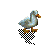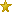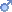# The Dink Network

## Auto indentation for code?

July 10th 2020, 08:02 PMtoofI disagree.
Can we please implement something like this? This really shouldn't be a hard script. This is what I've used for html version of DinkC to avoid going insane
```function formatCode() {
let elements = document.getElementsByTagName('pre');
for (let el of elements) {
let text = el.innerText;
let lines = text.match(/[^\r\n]+/g);
el.innerHTML = '';

lines.forEach((line, i) => lines[i] = line.trim());
lines = indentLines(lines);
lines.forEach(l => el.innerHTML += l);
}
}

function indentLines(lines) {
let indentSize = [' ', ' ', ' ', ' '];
let indentation = '';

lines.forEach((line, i) => {
if (line === '{') {
lines[i] = indentation + line;
indentSize.forEach(i => indentation += i);
}
else if (line === '}') {
indentSize.forEach(i => indentation = indentation.replace(i, ''));
lines[i] = indentation + line;
}
else {
lines[i] = indentation + line;
}
lines[i] += '\n';
lines[i] = fixVarName(lines[i]);
});

return lines;
}
function fixVarName(variable) {
return variable.replace(/¤t_sprite/, '&current_sprite');
}
```